# NCERT Solutions for Class 8 Maths Chapter 2 Exercise 2.4

NCERT Solutions for Class 8 Maths Chapter 2 Exercise 2.4 – Linear Equation in one variable, has been designed by the NCERT to test the knowledge of the student on the topic – Some More Applications of Linear Equation in one variable

### NCERT Solutions for Class 8 Maths Chapter 2 Exercise 2.4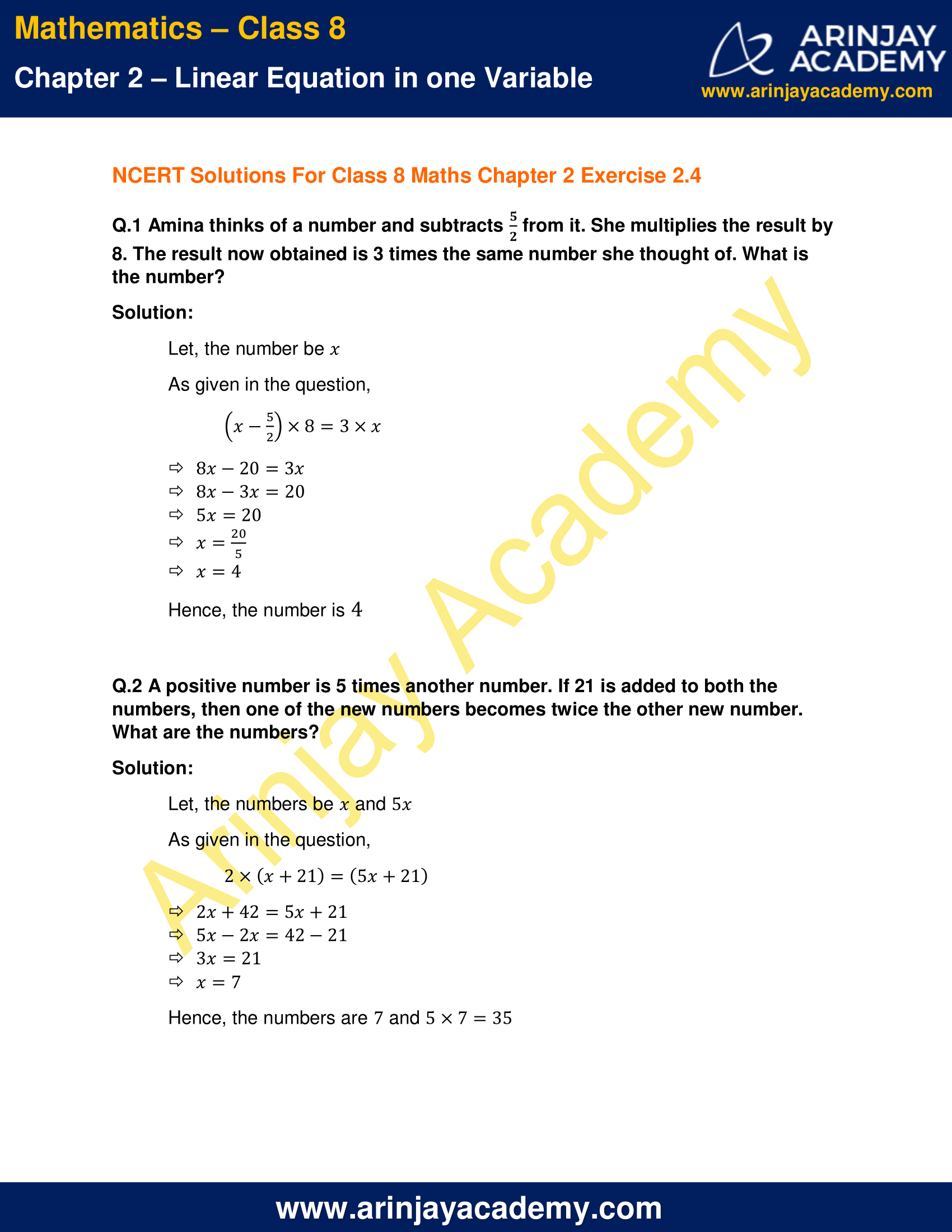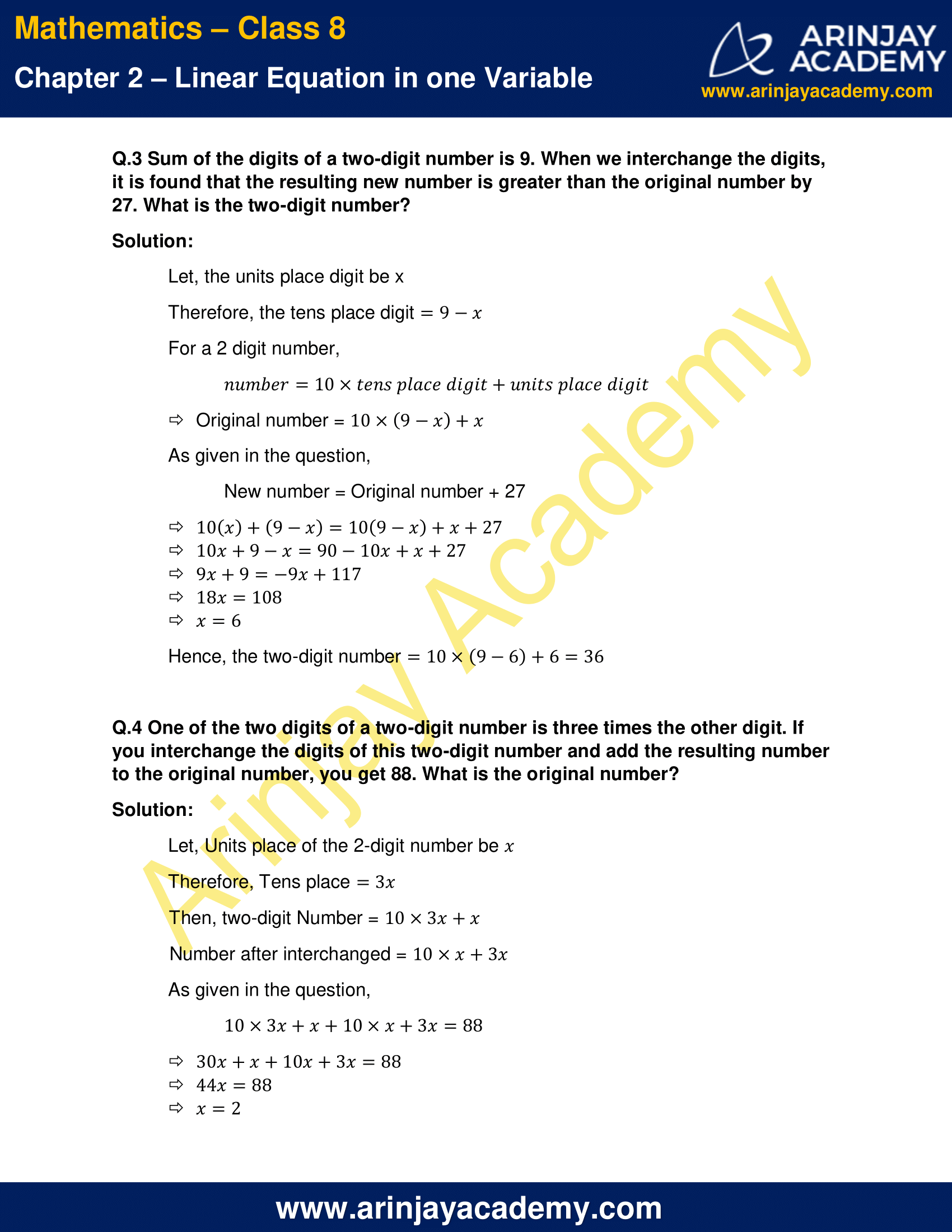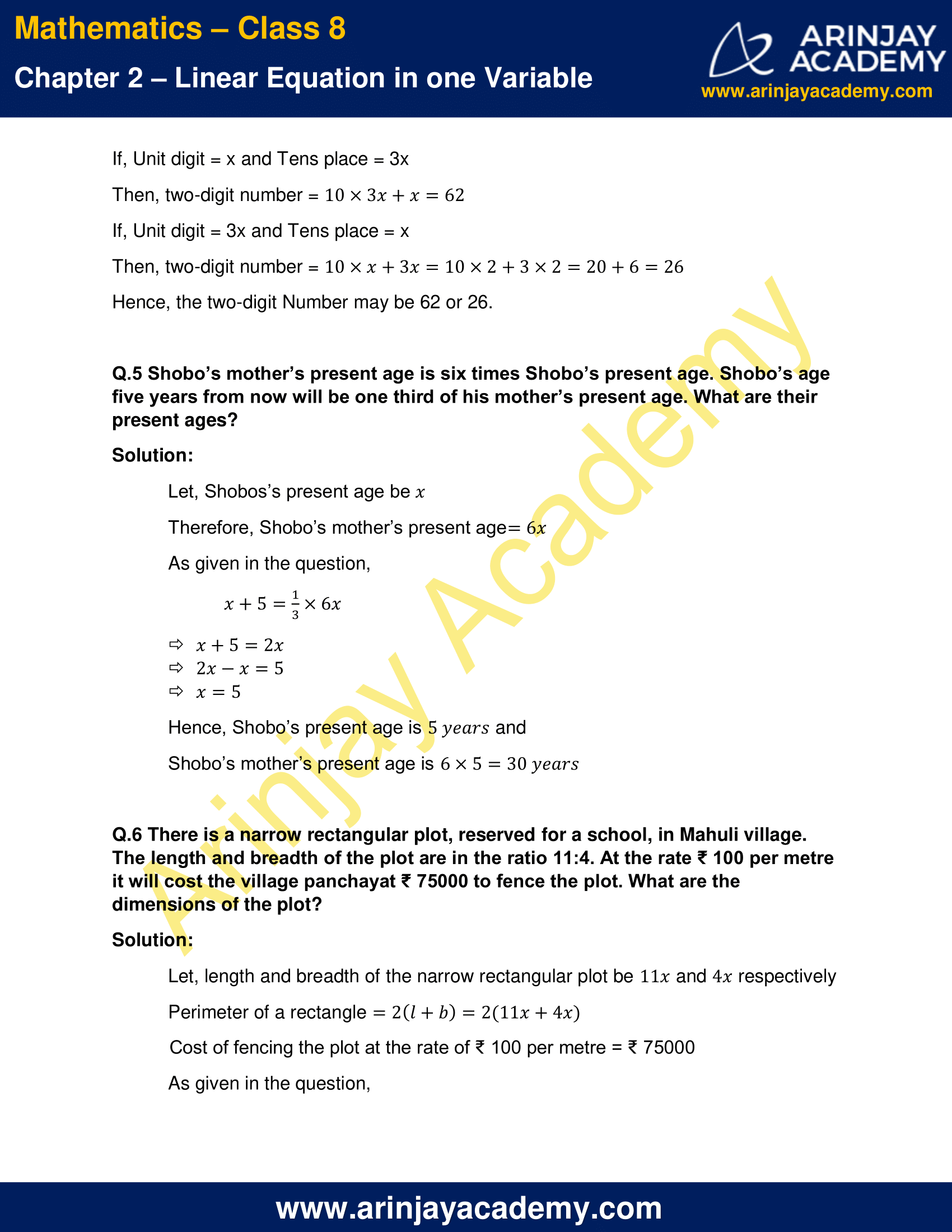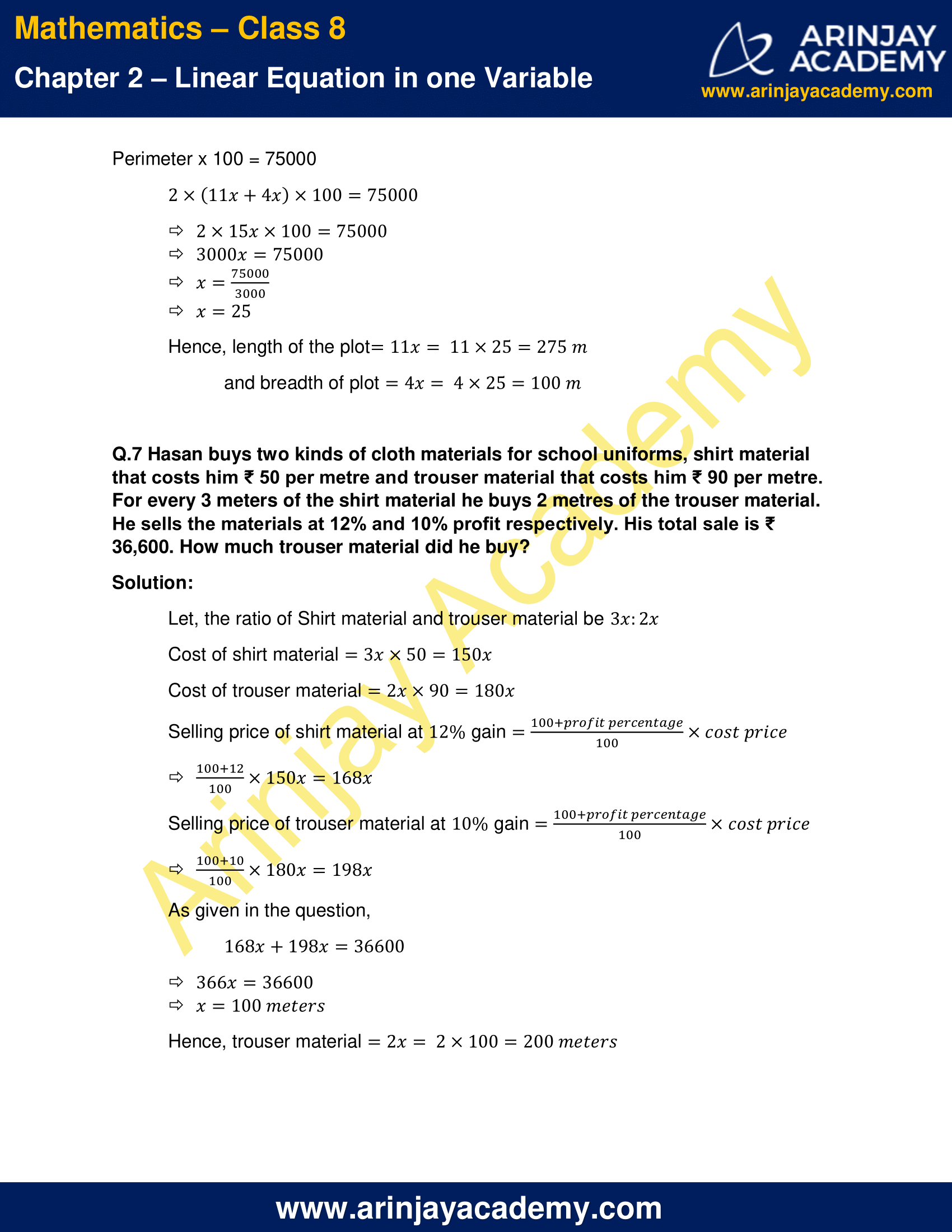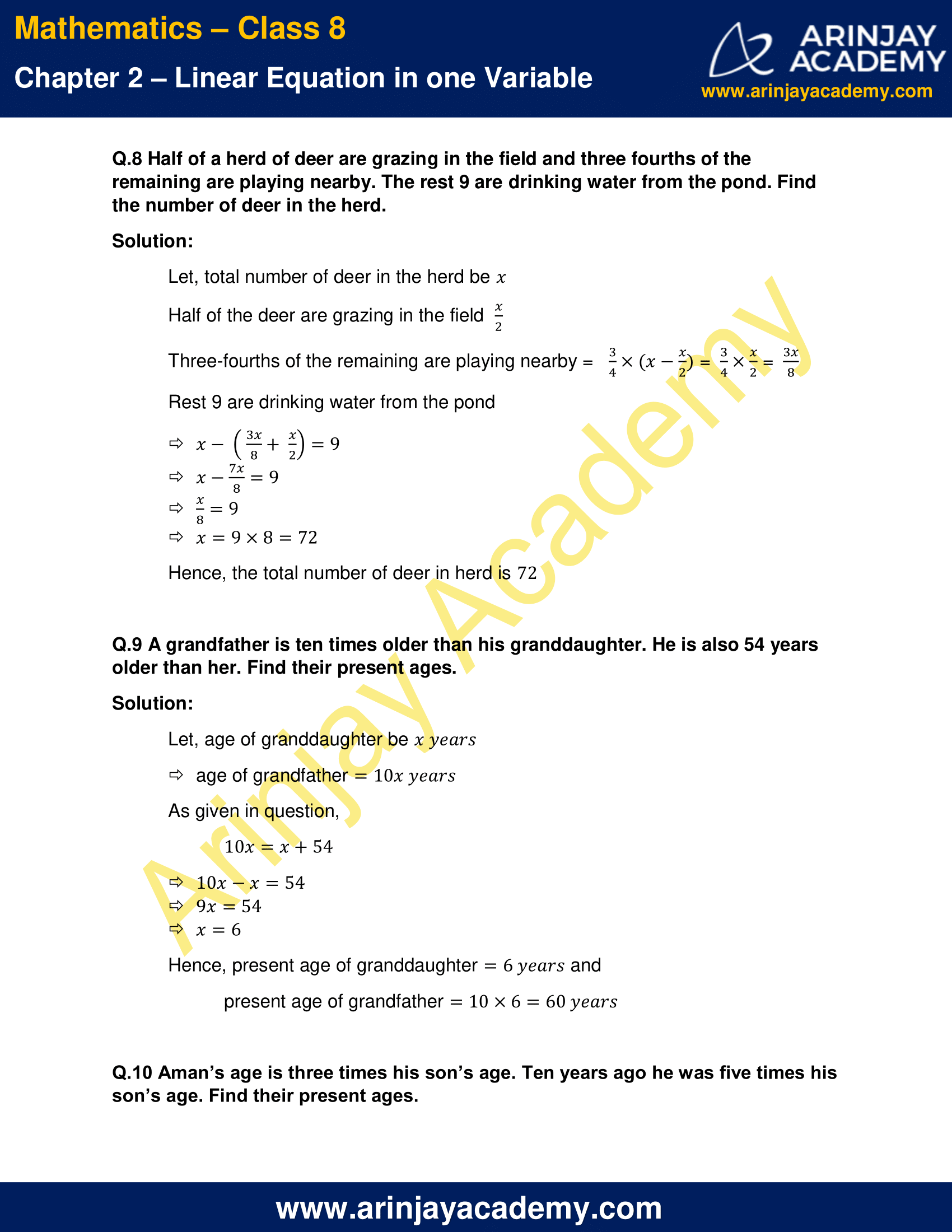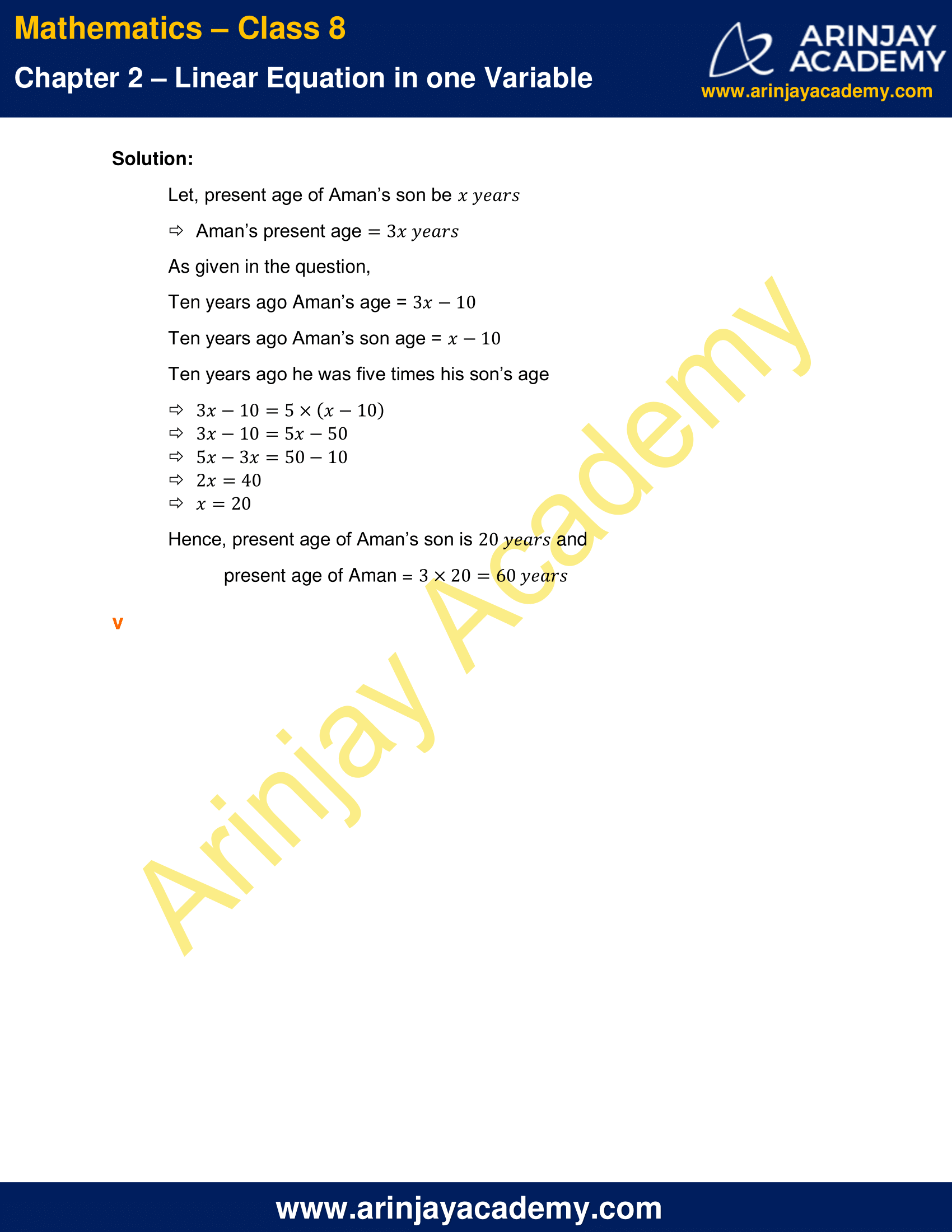NCERT Solutions for Class 8 Maths Chapter 2 Exercise 2.4

Q.1 Amina thinks of a number and subtracts 5/2 from it. She multiplies the result by 8. The result now obtained is 3 times the same number she thought of. What is the number?

Solution:

Let, the number be x
As given in the question,
[x – (5/2)] × 8 = 3 × x
8x – 20 = 3x
8x – 3x = 20
5x = 20
x = 20/5
x = 4
Hence, the number is 4

Q.2 A positive number is 5 times another number. If 21 is added to both the numbers, then one of the new numbers becomes twice the other new number. What are the numbers?

Solution:

Let, the numbers be x and 5x
As given in the question,
2 × (x+21) = (5x+21)
2x + 42 = 5x + 21
5x – 2x = 42 – 21
3x = 21
x = 7
Hence, the numbers are 7 and 5 x 7 = 35

Q.3 Sum of the digits of a two-digit number is 9. When we interchange the digits, it is found that the resulting new number is greater than the original number by 27. What is the two-digit number?

Solution:

Let, the units place digit be x
Therefore, the tens place digit = 9 – x
For a 2 digit number,
Number = 10 x tens place digit + units place digit
Original number = 10 × (9-x) + x
As given in the question,
New number = Original number + 27
10x + (9-x) = 10(9-x) + x + 27
10x + 9 – x = 90 – 10x + x + 27
9x + 9 = -9x + 117
18x = 108
x = 6
Hence, the two-digit number = 10 × (9-6) + 6 = 36

Q.4 One of the two digits of a two-digit number is three times the other digit. If you interchange the digits of this two-digit number and add the resulting number to the original number, you get 88. What is the original number?

Solution:

Let, Units place of the 2-digit number be x
Therefore, Tens place = 3x
Then, two-digit Number = 10 × 3x + x
Number after interchanged = 10 × x + 3x
As given in the question,
10 × 3x + x + 10 × x + 3x = 88
30x + x + 10x + 3x = 88
44x = 88
x = 2
If, Unit digit = x and Tens place = 3x
Then, two-digit number =10 × 3x + x = 62
If, Unit digit = 3x and Tens place = x
Then, two-digit number = 10 × x + 3x = 10 x 2 + 3 x 2 = 20 + 6 = 26
Hence, the two-digit Number may be 62 or 26.

Q.5 Shobo’s mother’s present age is six times Shobo’s present age. Shobo’s age five years from now will be one third of his mother’s present age. What are their present ages?

Solution:

Let, Shobos’s present age be x
Therefore, Shobo’s mother’s present age = 6x
As given in the question,
x+5 = (1/3) × 6x
x+5 = 2x
2x-x = 5
x = 5
Hence, Shobo’s present age is  5 years and Shobo’s mother’s present age is 6 x 5 = 30 years

Q.6 There is a narrow rectangular plot, reserved for a school, in Mahuli village. The length and breadth of the plot are in the ratio 11:4. At the rate ₹ 100 per metre it will cost the village panchayat ₹ 75000 to fence the plot. What are the dimensions of the plot?

Solution:

Let, length and breadth of the narrow rectangular plot be 11x and 4x respectively
Perimeter of a rectangle = 2(l+b) = 2(11x+4x)
Cost of fencing the plot at the rate of ₹ 100 per metre = ₹ 75000
As given in the question,
Perimeter x 100 = 75000
2 × (11x+4x) × 100 = 75000
2 × 15x × 100 = 75000
3000x = 75000
x = (75000/3000)
x = 25
Hence, length of the plot = 11x = 11 × 25 = 275m
and breadth of plot = 4x = 4 × 25 = 100m

Q.7 Hasan buys two kinds of cloth materials for school uniforms, shirt material that costs him ₹ 50 per metre and trouser material that costs him ₹ 90 per metre. For every 3 meters of the shirt material he buys 2 metres of the trouser material. He sells the materials at 12% and 10% profit respectively. His total sale is ₹ 36,600. How much trouser material did he buy?

Solution:

Let, the ratio of Shirt material and trouser material be 3x : 2x
Cost of shirt material = 3x × 50 = 150x
Cost of trouser material = 2x × 90 = 180x
Selling price of shirt material at 12% gain =(100+ Profit Percentage)/100 × Cost Price
= [(100+12)/100] × 150x
= 168x
Selling price of trouser material at 10% gain = (100 + Profit Percentage)/100 × Cost Price
= [(100+10)/100] × 180x
= 198x
As given in the question,
168x + 198x = 36600
366x = 36600
x = 100 meters
Hence, trouser material = 2x = 2 x 100 = 200 meters

Q.8 Half of a herd of deer are grazing in the field and three fourths of the remaining are playing nearby. The rest 9 are drinking water from the pond. Find the number of deer in the herd.

Solution:

Let, total number of deer in the herd be x
Half of the deer are grazing in the field (x/2)
Three-fourths of the remaining are playing nearby = (3/4) × [x – (x/2)] = (3/4) × (x/2) = (3x/8)
Rest 9 are drinking water from the pond
x – [(3x)/8 +(x/2)] = 9
x – (7x/8) = 9
(x/8) = 9
x = 9 × 8 = 72
Hence, the total number of deer in herd is 72

Q.9 A grandfather is ten times older than his granddaughter. He is also 54 years older than her. Find their present ages.

Solution:

Let, age of granddaughter be x years
Age of grandfather = 10x years
As given in question,
10x = x + 54
10x – x = 54
9x = 54
x = 6
Hence, present age of granddaughter = 6 years and present age of grandfather = 10 x 6 = 60 years

Q.10 Aman’s age is three times his son’s age. Ten years ago he was five times his son’s age. Find their present ages.

Solution:

Let, present age of Aman’s son be x years
Aman’s present age = 3x years
As given in the question,
Ten years ago Aman’s age = 3x – 10
Ten years ago Aman’s son age = x – 10
Ten years ago he was five times his son’s age
3x – 10 = 5(x-10)
3x – 10 = 5x – 50
5x – 3x = 50 – 10
2x = 40
x = 20
Hence, present age of Aman’s son is 20 years and present age of Aman = 3 x 20 = 60 years

The next Exercise for NCERT Solutions for Class 8 Maths Chapter 2 Exercise 2.5 – Linear Equation in one variable can be accessed by clicking here